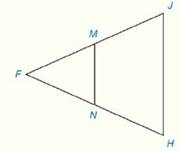Chapter 4.CR, Problem 23CRElementary Geometry For College St...

7th Edition
Alexander + 2 others
ISBN: 9781337614085

Solutions

Chapter
SectionElementary Geometry For College St...

7th Edition
Alexander + 2 others
ISBN: 9781337614085
Textbook Problem

Review ExercisesIn Review Exercises 22 to 24, M and N are the midpoints of F J - and F H - respectively.Given: J H = 12 m ∠ J = 80 ° m ∠ F = 60 ° Prove: M N ,   m ∠ F M N ,   m ∠ F N M

To determine

To Find:

The length of the line joining the mid points of the sides of the triangle and measure of angles based on the information provided.

Explanation

Formula Used:

Sum of the interior angle of a triangle is 180°.

Theorem on triangle:

The line segment that joins the midpoints of two sides of a triangle is parallel to the third side and has a length equal to one-half the length of the third side.

That is, ABC with midpoints M and N of AB- and AC-, respectively. Then MN-BC-. It can be concluded that BC=12(MN)

Calculation:

It is given that FJH is an isosceles triangle with M and N are the midpoints of FJ- and FH- respectively.

Also given that JH=12, mJ=80° and mF=60°.

Applying the theorem, MN is parallel to JH.

So mFHJ=mFNM and mFJH=mFMN.

Here mJ=80°

Still sussing out bartleby?

Check out a sample textbook solution.

See a sample solution

The Solution to Your Study Problems

Bartleby provides explanations to thousands of textbook problems written by our experts, many with advanced degrees!

Get Started

Find more solutions based on key concepts